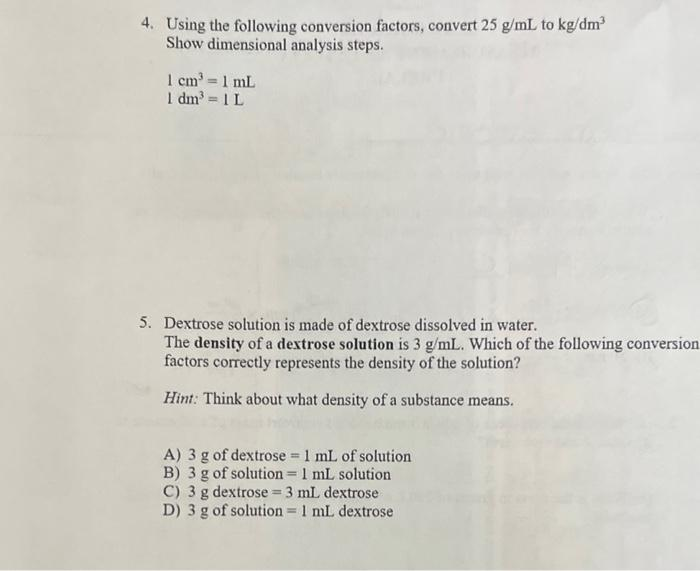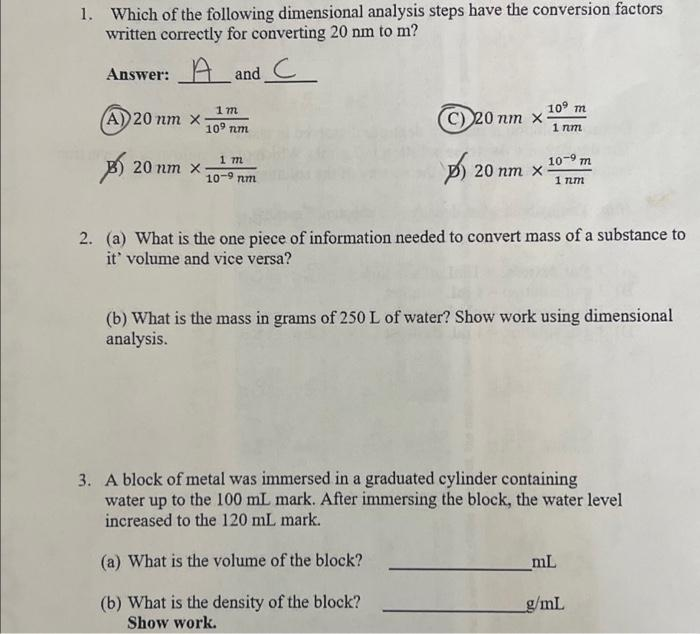Home / Expert Answers / Chemistry / need-help-with-full-work-shown-ans-clear-nbsp-4-using-the-following-conversion-factors-convert-pa498

# (Solved): need help with full work shown ans clear  4. Using the following conversion factors, convert $$... need help with full work shown ans clear4. Using the following conversion factors, convert \( 25 \mathrm{~g} / \mathrm{mL}$$ to $$\mathrm{kg} / \mathrm{dm}^{3}$$ Show dimensional analysis steps. $\begin{array}{l} 1 \mathrm{~cm}^{3}=1 \mathrm{~mL} \\ 1 \mathrm{dm}^{3}=1 \mathrm{~L} \end{array}$ 5. Dextrose solution is made of dextrose dissolved in water. The density of a dextrose solution is $$3 \mathrm{~g} / \mathrm{mL}$$. Which of the following conversion factors correctly represents the density of the solution? Hint: Think about what density of a substance means. A) $$3 \mathrm{~g}$$ of dextrose $$=1 \mathrm{~mL}$$ of solution B) $$3 \mathrm{~g}$$ of solution $$=1 \mathrm{~mL}$$ solution C) $$3 \mathrm{~g}$$ dextrose $$=3 \mathrm{~mL}$$ dextrose D) $$3 \mathrm{~g}$$ of solution $$=1 \mathrm{~mL}$$ dextrose 1. Which of the following dimensional analysis steps have the conversion factors written correctly for converting $$20 \mathrm{~nm}$$ to $$\mathrm{m}$$ ? Answer: $$A$$ and $$C$$ A) $$20 \mathrm{~nm} \times \frac{1 \mathrm{~m}}{10^{9} \mathrm{~nm}}$$ (C) $$20 \mathrm{~nm} \times \frac{10^{9} \mathrm{~m}}{1 \mathrm{~nm}}$$ B) $$20 \mathrm{~nm} \times \frac{1 \mathrm{~m}}{10^{-9} \mathrm{~nm}}$$ p) $$20 \mathrm{~nm} \times \frac{10^{-9} \mathrm{~m}}{1 \mathrm{~nm}}$$ 2. (a) What is the one piece of information needed to convert mass of a substance to it' volume and vice versa? (b) What is the mass in grams of $$250 \mathrm{~L}$$ of water? Show work using dimensional analysis. 3. A block of metal was immersed in a graduated cylinder containing water up to the $$100 \mathrm{~mL}$$ mark. After immersing the block, the water level increased to the $$120 \mathrm{~mL}$$ mark. (a) What is the volume of the block? $$\mathrm{mL}$$ (b) What is the density of the block? $$\mathrm{g} / \mathrm{mL}$$ Show work

We have an Answer from Expert